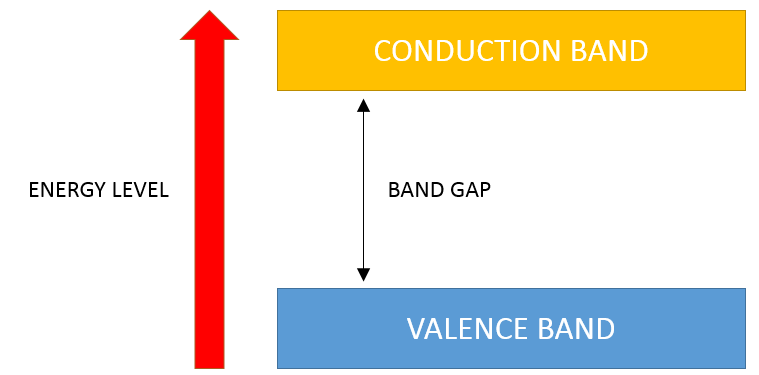#### Band Gap

In semiconducting materials, there is a conduction band and a valance band. The band gap is the energy range between the two bands where no electron states can exist.A high band gap means that the solar cell will produce a high voltage and low current. A low band gap means that the solar cell will product a low voltage and high current.

Since Power = Voltage x Current, there is an optimal band gap value for maximizing power. For solar cells, this value is 1.34 eV which results in a 33.7% efficiency.